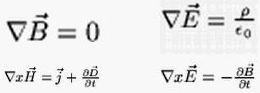Online JudgeProblem SetAuthorsOnline ContestsUser
Web Board
F.A.Qs
Statistical Charts
Problems
Submit Problem
Online Status
Prob.ID:
Register
Authors ranklist
Current Contest
Past Contests
Scheduled Contests
Award Contest
Register
Language:
Complicated Expressions
 Time Limit: 1000MS Memory Limit: 10000K Total Submissions: 1922 Accepted: 575

Description

The most important activity of ACM is the GSM network. As the mobile phone operator, ACM must build its own transmitting stations. It is very important to compute the exact behaviour of electro-magnetic waves. Unfortunately, prediction of electro-magnetic fields is a very complex task and the formulas describing them are very long and hard-to-read. For example, below are the Maxwell's Equations describing the basic laws of electrical engineering.ACM has designed its own computer system that can make some field computations and produce results in the form of mathematic expressions. Unfortunately, by generating the expression in several steps, there are always some unneeded parentheses inside the expression. Your task is to take these partial results and make them "nice" by removing all unnecessary parentheses.

Input

There is a single positive integer T on the first line of input. It stands for the number of expressions to follow. Each expression consists of a single line containing only lowercase letters, operators (+, -, *, /) and parentheses (( and )). The letters are variables that can have any value, operators and parentheses have their usual meaning. Multiplication and division have higher priority then subtraction and addition. All operations with the same priority are computed from left to right (operators are left-associative). There are no spaces inside the expressions. No input line contains more than 250 characters.

Output

Print a single line for every expression. The line must contain the same expression with unneeded parentheses removed. You must remove as many parentheses as possible without changing the semantics of the expression. The semantics of the expression is considered the same if and only if any of the following conditions hold:

• The ordering of operations remains the same. That means "(a+b)+c" is the same as "a+b+c", and "a+(b/c)" is the same as "a+b/c".
• The order of some operations is swapped but the result remains unchanged with respect to the addition and multiplication associativity. That means "a+(b+c)" and "(a+b)+c" are the same. We can also combine addition with subtraction and multiplication with division, if the subtraction or division is the second operation. For example, "a+(b-c)" is the same as "a+b-c".

You cannot use any other laws, namely you cannot swap left and right operands and you cannot replace "a-(b-c)" with "a-b+c".

Sample Input

```8
(a+(b*c))
((a+b)*c)
(a*(b*c))
(a*(b/c)*d)
((a/(b/c))/d)
((x))
(a+b)-(c-d)-(e/f)
(a+b)+(c-d)-(e+f)```

Sample Output

```a+b*c
(a+b)*c
a*b*c
a*b/c*d
a/(b/c)/d
x
a+b-(c-d)-e/f
a+b+c-d-(e+f)```

Source

[Submit]   [Go Back]   [Status]   [Discuss]Home PageGo BackTo top